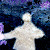# DC Current Measurement using Shunt Resistor

Designing of Digital Ammeter is basically a process of converting a voltmeter into Ammeter. We know that V=I x R. From this we can say that Voltage drop across resistor (shunt) is directly proportional to the current (V = I). In ammeter this resistance is called Shunt. Shunt resistance have very small value and it will not affect the load voltage. Most commonly 75mV shunts are available in market. Or you can use low value resistance.

#### What you will learn?

1. Where to connect shunt resistor to measure DC current?
2. What is shunt resistor?
3. Why it is having small value?
4. How arduino can be used to measure current using shunt resistor?
5. How to design amplifier circuit for shunt voltage?

## DC Current Measurement using Shunt Resistor Circuit

In this example we are measuring 50Amp current using 75mV shunt (Rsense). Shunt gives 75mV voltage across it when we pass the current of 50Amp. Arduino takes 5V as input for ADC, so the differential amplifier will convert 75mV to 5V.
Calculations for 50Amps 75mV Shunt
Vo is voltage given at ADC (A0) i.e. 5V
Rin = R1 = R3
Rf = R2 = R4
Vin = 75mV
Vo = Vin * (RF/Rin)

Where:
Vin = 75mV
Vo = 5V

Assume Rin=1K (You can assume Rin in the range of 1K to 50K)
Find Rin=?
Rin=R1=R3 = 66.66K
Rf=R2=R4 = 1K
You can use standard resistor and do reverse calculations in Arduino code

#### Components Required

1. 25Amps/75mVolts Shunt Resistor
2. Arduino Uno
3. Connecting Wires
4. OpAmp OP07
5. 66.66K, 1K Resistors

## Arduino Code for DC Current Measurement using Shunt Resistor

```/*
DC Current Measurement Using Shunt - www.circuits4you.com
Reads an analog input on pin 0, converts it to current, and prints the result to the serial monitor.
*/
const float ShuntAmps = 50;  //Enter the shunt maximum current

// the setup routine runs once when you press reset:
void setup() {
// initialize serial communication at 9600 bits per second:
Serial.begin(9600);
}

// the loop routine runs over and over again forever:
void loop() {
// read the input on analog pin 0:

// Convert the analog reading (which goes from 0 - 1023) to a Current (0 - 50):
float current = sensorValue * (ShuntAmps / 1024.0);

// print out the value you read:
Serial.print("Current:");
Serial.print(current);
Serial.println(" Amps");
delay(1000);
}
```

## Result of DC Current Measurement using Shunt Resistor

Open serial monitor and observe the current reading.

## One thought on “DC Current Measurement using Shunt Resistor”

1.Andrii Yaremych says:

Hi. There is an error in the article:

IF

Rin = 66.66K
Rf = 1K
Vin = 75mV

Vo = Vin * (RF/Rin)
Vo = 0.075V*1k/66k = 0.00114V

It should be
Vo = 0.075V*66k/1k = 4.95V

SO

Rf = R1 = R3 = 66.66K
Rin = R2 = R4 = 1K